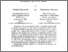# A recursive procedure for selecting optimal portfolio according to the MAD model

Michalowski W & Ogryczak W (1999). A recursive procedure for selecting optimal portfolio according to the MAD model. Control and Cybernetics 28 (4): 725-738.Preview
Text
A recursive procedure for selecting optimal portfolio according to the MAD model.pdf - Published Version

## Abstract

The mathematical model of portfolio optimization is usually represented as a bicriteria optimization problem where a reasonable trade-off between expected rate of return and risk is sought. In a classical Markowitz model the risk is measured by a variance, thus resulting in a quadratic programming model. As an alternative, the MAD model was proposed where risk is measured by (mean) absolute deviation instead of a variance. The MAD model is computationally attractive, since it is transformed into an easy to solve linear programming program. In this paper we present a recursive procedure which allows to identify optimal portfolio of the MAD model depending on investor's downside risk aversion.

Item Type: Article Downside risk aversion; Linear programming; Portfolio optimization Luke Kirwan 04 Aug 2016 08:58 04 Aug 2016 08:58 http://pure.iiasa.ac.at/13569View Item